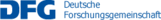## A02 –  Classical and quantum kinetic equations

The kinetic theory of gases studies the behavior of interacting particle systems which evolve by Hamiltonian dynamics. This theory is applicable in dilute systems in which some macroscopic characteristic length is of the same order of magnitude as the mean free path between particle collisions. This project aims to study two classes of problems arising from kinetic theory, namely the rigorous derivation of kinetic equations taking as starting point interacting particle systems (both Hamiltonian and stochastic) and the qualitative analysis of the behavior of their solutions. In the two previous funding periods we studied the qualitative behavior of solutions of kinetic equations modeling classical and quantum gases. We also acquired expertise in the derivation of kinetic models starting from particle systems. Some of the problems that we plan to study are as follows. We want to obtain a rigorous derivation of the homogeneous quantum Boltzmann equation for bosons from a stochastic particle model that takes into account the Bose-Einstein statistics. We are also interested in the study of Lorentz gases, in which one tagged particle interacts with a random background of fixed scatterers, or Rayleigh gases in the case of moving scatterers. We want to address rigorously the problem of the derivation of kinetic equations describing the evolution of a tagged particle which interacts with a distribution of scatterers by means of long-range interactions, or more precisely potentials behaving as Coulombian potentials at long distances. During the last funding period we began the study of a particular class of solutions of the Boltzmann equation, known as homoenergetic solutions, which give insight about the dynamics of open systems. During the next funding period we plan to continue the analysis of the mathematical properties of these solutions, including their stability. We also would like to apply the kinetic point of view to other problems of statistical physics, namely the geometry of semiflexible polymer chains and the distribution of energy of some systems of weakly interacting waves. We will analyze the properties of the solutions of the resulting equations.

Name Institute Location Phone
Velázquez, Juan JoséIAMEn60/2.02362378velazquez@iam.uni-bonn.de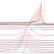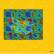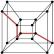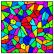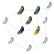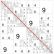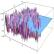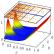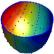## Contact

Coordinator: Prof. Dr. Stefan Müller